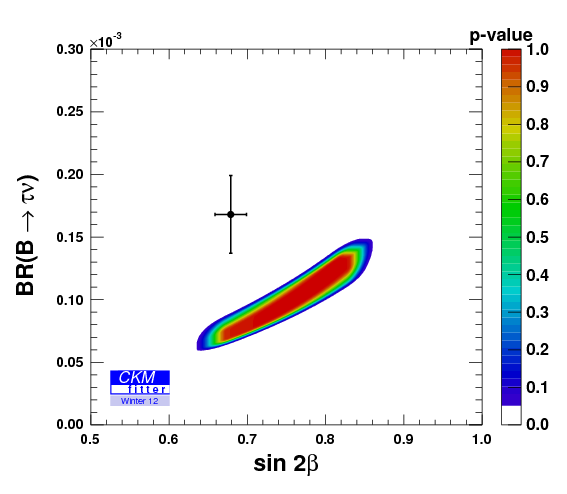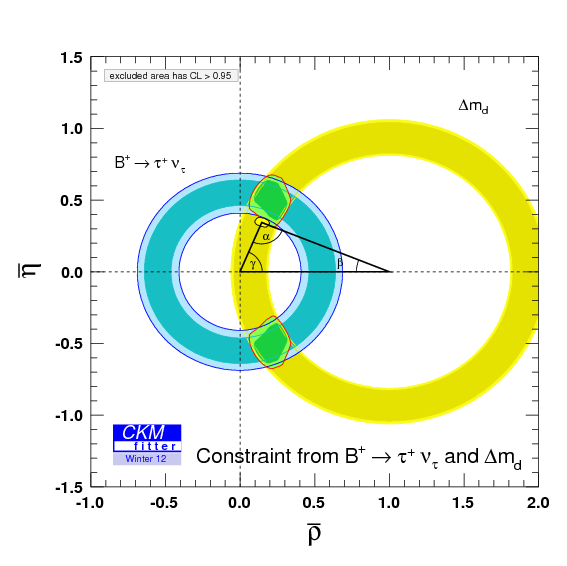## View Blog | Read Bio

### How to discover new physics

The biggest news at CIPANP 2012 for particle physicists seems to be coming from the “low” energy frontier, at energies in the ballpark of 10GeV and lower. This may come as a surprise to some people, after all we’ve had experiments working at these energies for a few decades now, and there’s a tendency to think that higher energies mean more potential for discovery. The lower energy experiments have a great advantage over the giants at LHC and Tevatron, and this is richer collection of analyses.

There’s a big difference between discovering a new phenomenon and discovering new physics, which is something that most people (including physicists!) don’t appreciate enough. Whenever a claim of new physics is made we need to look at the wider implications of the idea. For example, let’s say that we see the decay of a $$\tau$$ lepton to an proton and a $$\pi^0$$ meson. The Feynman diagram would look something like this:tau lepton decay to a proton and a neutral pion, mediated by a leptoquark

The “X” particle is a leptoquark, and it turns leptons into quarks and vice versa. Now for this decay to happen at an observable rate we need something like this leptoquark to exist. There is no Standard Model process for $$\tau\to p\pi^0$$ since it violates baryon number (a process which is only allowed under very special conditions). So suppose someone claims to see this decay, does this mean that they’ve discovered new physics? The answer is a resounding “No”, because if they make a claim of new physics they need to look elsewhere for similar effects. For example, if the leptoquark existed the proton could decay with this process:

We have very stringent tests on the lifetime of the proton, and the lower limits are currently about 20 orders of magnitude longer than the age the universe. Just take a second to appreciate the size of that limit on the lifetime. The proton lasts for at least 20 orders of magnitude longer than the age of the universe itself. So if someone is going to claim that they have proven the leptoquark exists we need to check that what they have seen is consistent with the proton lifetime measurements. A claim of new physics is stronger than a claim of a new phenomena, because it must be consistent with all the current data, not just the part we’re working.

How does all this relate to CIPANP 2012 and the low energy experiments? Well it turns out that there are a handful of large disagreements in this regime that all tend to involve the same particles. The $$B$$ meson can decay to several lighter particles and the BaBar experiment has seen the decays to the $$\tau$$ lepton are higher than they should be. The disagreement is more than $$3\sigma$$ disagreement with the Standard Model predictions for $$B\to D^{(*)}\tau\nu$$, which is interesting because it involves the heaviest quarks in bound states, and the heaviest lepton. It suggests that if there is a new particle or process, that it favors coupling to heavy particles.Standard model decays of the B mesons to τν, Dτν, and D*τν final states

In another area of $$B$$ physics we find that the branching fraction $$\mathcal{B}(B\to\tau\nu)$$ is about twice as large as we expect from the Standard Model. You can see the disagreement in the following plot, which compares two measurements ($$\mathcal{B}(B\to\tau\nu)$$ and $$\sin 2\beta$$) to what we expect given everything else. The distance between the data point and the most favored region (center of the colored region) is very large, about $$3\sigma$$ in total!The disagreement between B→τν, sin2β and the rest of the unitary triangle measurements (CKMFitter)

Theorists love to combine these measurements using colorful diagrams, and the best known example is the unitary triangle. If the CKM mechanism describes all the quark mixing processes then all of the measurements should agree, and they should converge on a single apex of the triangle (at the angle labeled $$\alpha$$). Each colored band corresponds to a different kind of process, and if you look closely you can see some small disagreements between the various measurements:

The blue $$\sin 2\beta$$ measurement is pulling the apex down slightly, and green $$|V_{ub}|$$ measurement is pulling it in the other direction. This tension shows some interesting properties when we try to investigate it further. If we remove the $$\sin 2\beta$$ measurement and then work out what we expect based on the other measurements, we find that the new “derived” value of $$\sin 2\beta$$ is far off what is actually measured. The channel used for analysis of $$\sin 2\beta$$ is often called the golden channel, and it has been the main focus of both BaBar and Belle experiments since their creation. The results for $$\sin2\beta$$ are some of the best in the world and they have been checked and rechecked, so maybe the problem is not associated with $$\sin 2\beta$$.

Moving our attention to $$|V_{ub}|$$ the theorists at CKMFitter decided to split up the contributions based on the semileptonic inclusive and exclusive decays, and from $$\mathcal{B}(B\to\tau\nu)$$. When this happens we find that the biggest disagreement comes from $$\mathcal{B}(B\to\tau\nu)$$ compared to the rest. The uncertainties get smaller when $$\mathcal{B}(B\to\tau\nu)$$ is combined with the $$B$$ mixing parameter, $$\Delta m_d$$, which is well understood in terms of top quark interactions, but these results still disagree with everything else!:Disagreement between B→τν, Δmd and the rest of the unitary triangle measurments (CKMFitter)

What this is seeming to tell us is that there could be a new process that affects $$B$$ meson interactions, enhancing decays with $$\tau$$ leptons in the final state. If this is the case then we need to look at other processes that could be affected by these kinds of processes. The most obvious signal to look for at the LHC is something like production of $$b$$ quarks and $$\tau$$ leptons. Third generation leptoquarks would be a good candidate, as long as they cannot mediate proton decay in any way. Searching for a new particle of a new effect is the job of the experimentalist, but creating a model that accommodates the discoveries we make is the job of a theorist.

That, in a nutshell is the difference between discovering a new phenomenon and discovering new physics. Anyone can find a bump in a spectrum, or even discover a new particle, but forming a consistent model of new physics takes a long time and a lot of input from all different kinds of experiments. The latest news from BaBar, Belle, CLEO and LHCb are giving us hints that there is something new lurking in the data. I can’t wait to see wait to see what our theorist colleagues do with these measurements. If they can create a model which explains anomalously high branching fractions $$\mathcal{B}(B\to\tau\nu)$$, $$\mathcal{B}(B\to D\tau\nu)$$, and $$\mathcal{B}(B\to D^*\tau\nu)$$, which tells us where else to look then we’re in for an exciting year at LHC. We could see something more exciting than the Higgs in our data!

(CKMFitter images kindly provided by the CKMfitter Group (J. Charles et al.), Eur. Phys. J. C41, 1-131 (2005) [hep-ph/0406184], updated results and plots available at: http://ckmfitter.in2p3.fr)

Tags: , , , ,